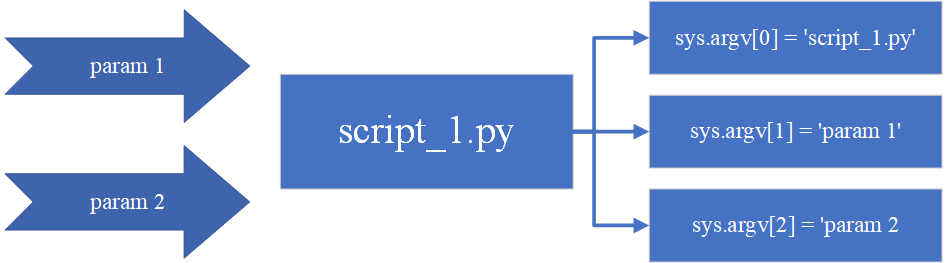# Python 命令行参数详解

### Python 命令行参数详解

• 0. 命令行参数
• 1. sys.argv
• 2. getopt
• 2.1 getopt.getopt 方法
• 2.2 Exception getopt.GetoptError
• 3. argparse
• ## 0. 命令行参数

通常，对于大型项目程序而言，执行程序的一个必要的步骤是正确处理命令行参数，这些命令行参数是提供给包含某种参数化信息的程序或脚本的参数。例如，在计算机视觉项目中，图像和不同类型的文件通常作为命令行参数传递给脚本，用于使程序可以处理不同图片或者不同类型文件。
命令行参数是参数化程序执行的一种常见且简单的方法，下面主要介绍三种常见的获取和解析命令行参数的方法。

## 1. sys.argv

为了处理命令行参数，Python 中内置了 `sys.argv` 模块，通过模块中的 `sys.argv` 就可以访问到所有的命令行参数，它的返回值是包含所有命令行参数的列表 (list)。当程序执行时，Python 从命令行获取所有值并将它们存储在 sys.argv 列表中。列表的第一个元素 `sys.argv` 是脚本的完整路径(或脚本名称——取决于具体操作系统)。列表的第二个元素是脚本的第一个命令行参数，即 `sys.argv`，依此类推。这可以通过下图中清晰的看出，其中 `script_1.py` 脚本使用两个参数执行：接下来，让我们看看 `sys.argv` 是如何工作的，首先编写 `scripy_1.py` 脚本：

``````import sys
print("正在运行的脚本名称: '{}'".format(sys.argv))
print("脚本的参数数量: '{}'".format(len(sys.argv)))
print("脚本的参数: '{}'".format(str(sys.argv)))
``````

如果我们不使用任何参数执行这个脚本：

``````python script_1.py
``````

将会看到如下信息：

``````正在运行的脚本名称: 'script_1.py'
脚本的参数数量: '1'
脚本的参数: '['script_1.py']'
``````

如果我们使用多个参数执行此脚本:

``````python script_1.py OpenCV -i test.png
``````

将得到以下信息：

``````正在运行的脚本名称: 'script_1.py'
脚本的参数数量: '4'
脚本的参数: '['script_1.py', 'OpenCV', '-i', 'test.png']'
``````

如上所示，列表的第一个元素 script_1.py (sys.argv) 是脚本名称。列表的第二个元素 (sys.argv) OpenCV 是脚本的第一个参数。但同时也可以看到，sys.argv 将命令行选项 `-i` 也识别为参数，这样并不能方便的满足我们的需求，因此引入 `getopt` 模块来识别命令行选项。

## 2. getopt

`getopt` 模块是专门处理命令行参数的模块，用于获取命令行选项和参数。命令行选项使得程序的参数更加灵活，其支持短选项模式(-)和长选项模式(–)。
该模块提供了两个方法及一个异常处理来解析命令行参数。

### 2.1 getopt.getopt 方法

`getopt.getopt` 方法用于解析命令行参数列表，其语法格式如下：

``````getopt.getopt(args, options[, long_options])
``````

方法参数说明如下表所示：

参数 说明
args 要解析的命令行参数列表，一般是sys.argv[1:]，需要过滤掉脚本名(sys.argv)
options 以字符串的格式定义，options 后的冒号 “`:`” 表示如果设置该选项，必须有附加的参数，否则就不附加参数
long_options 以列表的格式定义，long_options 后的等号 “`=`” 表示该选项必须有附加的参数，不带冒号表示该选项不附加参数

该方法返回值由两个元素组成: 第一个是 (option, value) 元组的列表。 第二个是参数列表，包含那些没有 – 或 – 的参数。
下面编写 `script_2.py` 脚本进行演示：

``````import sys
import getopt

def main(argv):
input_file = ""
output_file = ""
# "hi:o:": 短格式分析串, h 后面没有冒号, 表示后面不带参数; i 和 o 后面带有冒号, 表示后面带参数
# ["help", "input_file=", "output_file="]: 长格式分析串列表, help后面没有等号, 表示后面不带参数; input_file和output_file后面带冒号, 表示后面带参数
# 返回值包括 `opts` 和 `args`, opts 是以元组为元素的列表, 每个元组的形式为: (选项, 附加参数)，如: ('-i', 'test.png');
# args是个列表，其中的元素是那些不含'-'或'--'的参数
opts, args = getopt.getopt(argv[1:], "hi:o:", ["help", "input_file=", "output_file="])

for opt, arg in opts:
if opt in ("-h", "--help"):
print('script_2.py -i <input_file> -o <output_file>')
print('or: test_arg.py --input_file=<input_file> --output_file=<output_file>')
sys.exit()
elif opt in ("-i", "--input_file"):
input_file = arg
elif opt in ("-o", "--output_file"):
output_file = arg
print('输入文件为：', input_file)
print('输出文件为：', output_file)

# 打印不含'-'或'--'的参数
for i in range(0, len(args)):
print('不含'-'或'--'的参数 %s 为：%s' % (i + 1, args[i]))

if __name__ == "__main__":
main(sys.argv)
``````

使用带有命令行选项的命令执行此脚本，以下两种方式是等价的：

``````# 方式1
python scripy_1.py -i test.png -o output.png OpenCV
# 方式2
python scripy_1.py --input_file test.png --output_file output.png OpenCV
``````

输出得到以下信息：

``````输入文件为： test.png
输出文件为： output.png
不含'-'或'--'的参数 1 为：OpenCV
``````

### 2.2 Exception getopt.GetoptError

在参数列表中没有找到所传递参数，或选项的需要的参数为空时会触发该异常。异常的参数是一个字符串，表示错误的原因。属性 `msg``opt` 为相关选项的错误信息。
在上述代码中添加异常处理，检查此错误信息：

``````# ...
def main(argv):
input_file = ""
output_file = ""
try:
opts, args = getopt.getopt(argv[1:], "hi:o", ["help", "input_file=", "output_file="])
except getopt.GetoptError as e:
print(e.msg)
print(e.opt)
sys.exit(2)
# ...
``````

使用错误的格式选项传递参数执行脚本：

``````python scripy_1.py -f
``````

输出以下错误信息：

``````option -f not recognized
f
``````

## 3. argparse

当程序中使用采用复杂参数或多个文件名时，推荐使用 Python 的 `argparse` 库，它以系统的方式处理命令行参数，从而可以编写用户友好的命令行程序。Python 标准库 argparse 同样也是用于解析命令行参数的模块。首先，由程序确定所需的参数，然后， `argparse` 将这些参数解析为 `sys.argv`。此外，`argparse` 会生成帮助和使用信息提示，并在提供无效参数时发出错误。
为了介绍此模块，编写 `script_3.py`，如下所示：

``````import argparse
parser = argparse.ArgumentParser()
parser.parse_args()
``````

不带参数运行此脚本不会向 `stdout` 显示任何内容。但是，如果使用 `--help``-h` 选项，将得到脚本的使用信息提示：

``````usage: scripy_3.py [-h]
optional arguments:
-h, --help show this help message and exit
``````

指定其他参数会导致错误，例如使用如下命令：

``````scripy_3.py -i
``````

则会报导致错误：

``````usage: scripy_3.py [-h]
argparse_minimal.py: error: unrecognized arguments: -i
``````

由于未定义参数，因此不允许其他参数，接下来就添加一个参数，编写 `script_4.py` 脚本：

``````import argparse
parser = argparse.ArgumentParser()
parser.add_argument("first_argument", help="this is the string text in connection with first_argument")
args = parser.parse_args()
print(args.first_argument)
``````

这里添加了 `add_argument()` 方法。此方法用于指定程序将接受哪些命令行选项，此处添加了 `first_argument` 参数。此外， `argparse` 模块存储所有参数，将其名称与每个添加参数的名称相匹配——在此处为 first_argument 。为了获得参数值，需要使用 `args.first_argument`
如果此脚本以下示方法执行，则输出为 10：

``````python scripy_4.py 10
``````

但如果脚本在没有参数的情况下执行，则将输出以下信息：

``````usage: scripy_4.py [-h] first_argument
scripy_4.py: error: the following arguments are required: first_argument
``````

最后，如果我们使用 `-h` 选项执行脚本，输出将如下所示：

``````usage: scripy_4.py [-h] first_argument

positional arguments:
first_argument  this is the string text in connection with first_argument

optional arguments:
-h, --help      show this help message and exit
``````

默认情况下，argparse 将提供的选项视为字符串。因此，如果参数不是字符串，则应使用 `type` 选项。使用 `script_5.py` 脚本，其中添加了两个参数，这两个参数是 `int` 类型：

``````import argparse
parser = argparse.ArgumentParser()
parser.add_argument("first_number", help="first number to be added", type=int)
parser.add_argument("second_number", help="second number to be added", type=int)
args = parser.parse_args()
print("args: '{}'".format(args))
print("the sum is: '{}'".format(args.first_number + args.second_number))
args_dict = vars(parser.parse_args())
print("args_dict dictionary: '{}'".format(args_dict))
print("first argument from the dictionary: '{}'".format(args_dict["first_number"]))
``````

在前面的示例中，通过调用 `vars()` 函数将参数存储在字典中：

``````args_dict = vars(parser.parse_args())
print("args_dict dictionary: '{}'".format(args_dict))
print("first argument from the dictionary: '{}'".format(args_dict["first_number"]))
``````

如果不带参数执行脚本：

``````python script_5.py
``````

则输出如下：

``````usage: scripy_5.py [-h] first_number second_number
scripy_5.py: error: the following arguments are required: first_number, second_number
``````

此外，如果我们使用 -h 选项执行脚本：

``````python script_5.py --help
``````

输出将如下所示：

``````usage: scripy_1.py [-h] first_number second_number

positional arguments:
first_number   first number to be added
second_number  second number to be added

optional arguments:
-h, --help     show this help message and exit
``````

如果此脚本以如下方式执行：

``````python script_5.py 123 456
``````

则输出如下：

``````args: 'Namespace(first_number=123, second_number=456)'
the sum is: '579'
args_dict dictionary: '{'first_number': 123, 'second_number': 456}'
first argument from the dictionary: '123'
``````

更多 `argparse` 的高级介绍可以在官方文档中看到，其中包括了大量示例

来源：盼小辉丶

物联沃分享整理
物联沃-IOTWORD物联网 » Python 命令行参数详解